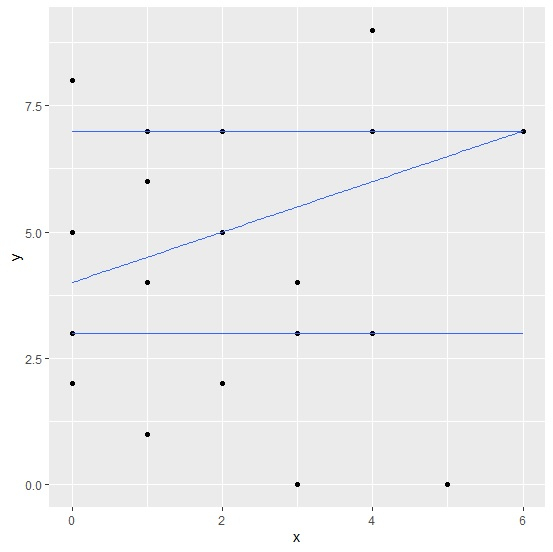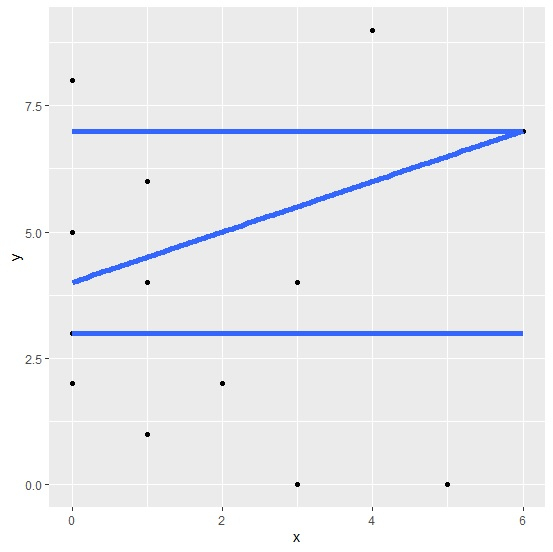# How to create quantile regression plot with larger width of lines using ggplot2 in R?

To create quantile regression plot with larger width of lines using ggplot2 in R, we can follow the below steps −

• First of all, create a data frame.

• Then, use stat_quantile function with size argument and geom_point function of ggplot2 package to create quantile regression plot.

## Example

#### Create the data frame

Let’s create a data frame as shown below −

x<-rpois(25,2)
y<-rpois(25,5)
df<-data.frame(x,y)
df

## Output

On executing, the above script generates the below output(this output will vary on your system due to randomization) −

   x y
1  1 6
2  1 1
3  3 4
4  4 9
5  4 7
6  2 5
7  1 7
8  1 4
9  2 7
10 3 3
11 4 3
12 2 5
13 4 7
14 2 7
15 0 8
16 5 0
17 3 4
18 0 2
19 3 0
20 0 5
21 2 5
22 2 5
23 6 7
24 2 2
25 0 3

Create quantile regression plot with larger width of lines

Using stat_quantile function with size argument and geom_point function of ggplot2 package to create quantile regression plot for the data stored in data frame df −

x<-rpois(25,2)
y<-rpois(25,5)
df<-data.frame(x,y)
library(ggplot2)

ggplot(df,aes(x,y))+geom_point()+stat_quantile(formula=y~x,quantiles=c(0.25,0.50,0.75
))

## OutputCreate quantile regression plot with larger width of lines

Using stat_quantile function with size argument and geom_point function of ggplot2 package to create quantile regression plot with larger width of lines for the data stored in data frame df −

x<-rpois(25,2)
y<-rpois(25,5)
df<-data.frame(x,y)
library(ggplot2)
ggplot(df,aes(x,y))+geom_point()+stat_quantile(formula=y~x,quantiles=c(0.25,0.50,0.75
),size=2)

## Output